In :
%load_ext autoreload
import matplotlib
import matplotlib.pyplot as plt
%matplotlib inline
from numpy.random import uniform, exponential
import numpy as np
import pandas as pd
import seaborn as sb
from lifelines.utils import survival_table_from_events

## plot/figure defaults
plt.style.use('ggplot')
matplotlib.rcParams['figure.figsize'] = (15.0, 8.0)
plt.rcParams['font.size'] = 20
plt.rcParams['axes.labelsize'] = 13
plt.rcParams['axes.labelweight'] = 'bold'
plt.rcParams['axes.titlesize'] = 13
plt.rcParams['xtick.labelsize'] = 13
plt.rcParams['ytick.labelsize'] = 13
plt.rcParams['legend.fontsize'] = 13
plt.rcParams['figure.titlesize'] = 'large'


# Survival Modeling in Stan¶

-- TODO insert image here

# a very brief introduction¶

Survival analysis typically involves time to event as the outcome of interest

Typical applications include:

• biomedical research (time to clinical event or death)
• digital marketing (time to click or KPI)
• machine (time to mechanical failure)
• psychology (e.g. studies of delayed gratification)
• ... etc

## key terms¶

• censoring: when an event isn't observed, but we know
• it happened after time b (right censoring)
• it happened before time a (left censoring)
• it happened between times a and b (interval censoring)

Survival analysis typically makes an assumption of non-informative censoring.

If this assumption holds, it means that the true event time (if it were to be observed) is unrelated to the censored time.

In a context of a well-designed clinical trial, this assumption is addressed by the study design which imposes an independent censoring process.

For example, it's not uncommon for follow-up time to be truncated after a predetermined time $t=10$ for all patients.

In :
# (prep for example - borrowed from http://lifelines.readthedocs.io/en/latest/Survival%20Analysis%20intro.html)
from numpy.random import uniform, exponential
import numpy as np

## example of censored data
def plot_example():
N = 25
current_time = 10

actual_lifetimes = np.array([[exponential(12), exponential(5)][uniform()<0.3] for i in range(N)])
fig = plt.figure()
_ = plt.xlim(0, 25)
_ = plt.vlines(10, 0, 30, lw=2, linestyles="--")
_ = plt.xlabel('time')
_ = plt.title('Births and deaths of a hypothetical population, censored at $t=10$', size=20)

In :
plot_example()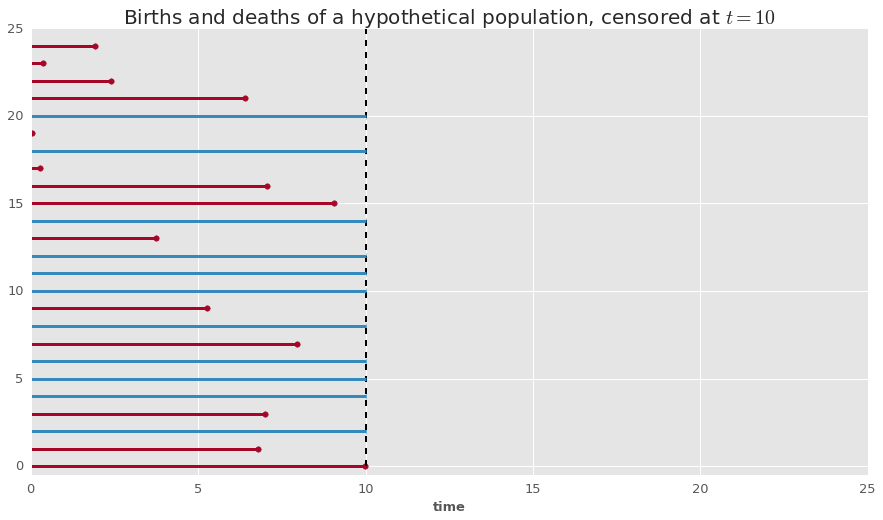however, this assumption can sometimes be violated if, for example, less healthy patients are more likely to drop out of the study early.

## key terms (cont'd)¶

• failure event: the outcome event of interest
• survival: not failure
• hazard: risk for failure events

Survival

We first define a Survival function $S$ as the probability of surviving to time $t$:

$$S(t)=Pr(Y > t)$$

where $T$ is the true survival time.

hazard

We also define the instantaneous hazard function $\lambda$ as the probability of a failure event occuring in the interval [$t$, $t+\delta t$], given survival to time $t$:

$$\lambda(t) = \lim_{\delta t \rightarrow 0 } \; \frac{Pr( t \le Y \le t + \delta t | Y > t)}{\delta t}$$

Which is equal to

$$\lambda(t) = \frac{-S'(t)}{S(t)}$$

Solving this

$$\lambda(t) = \frac{-S'(t)}{S(t)}$$

yields the following:

$$S(t) = \exp\left( -\int_0^t \lambda(z) dz \right)$$

which provides a useful way for us to switch from modeling hazards to modeling Survival.

The integral in this equation is also sometimes called the cumulative hazard, here noted as $H(t)$.

$$H(t) = \int_0^t \lambda(z) dz$$

It's worth pointing out that, by definition, the cumulative hazard (estimating $Pr(Y \lt t)$) is the complementary c.d.f of the Survival function (which estimates $Pr(Y \ge t)$).

Let's consider a simple hazard function $\lambda(t)$ as constant over time.

$$\lambda(t) = a$$

Cumulative hazard ($H$) would be:

$$H(t) = \int_0^t \lambda(z) dz = at$$

And the Survival function would be:

$$S(t) = \exp\left( -\int_0^t \lambda(z) dz \right) = \exp ( − a t )$$

Graphically, this would look like the following:

In :
## prep example plot for hazard <> Survival curves
def plot_survival_exp(N, censor_time, rate):

sample_data = pd.DataFrame({
't': np.linspace(0, censor_time, num=N),
})
sample_data['hazard'] = rate
sample_data['cum_hazard'] = rate*sample_data.t
sample_data['Survival'] = np.exp(-1 * rate * sample_data.t)
fig = plt.figure()
_ = plt.subplot(131)
_ = plt.plot(sample_data.t, sample_data.hazard, 'b')
_ = plt.title('Hazard $\lambda(t)$', size=20)
_ = plt.xlabel('time')
_ = plt.subplot(132)
_ = plt.plot(sample_data.t, sample_data.cum_hazard, 'r')
_ = plt.title('Cum. hazard $H(t)$', size=20)
_ = plt.xlabel('time')
_ = plt.subplot(133)
_ = plt.plot(sample_data.t, sample_data.Survival, 'g')
_ = plt.title('Survival $S(t)$', size=20)
_ = plt.xlabel('time')


In :
plot_survival_exp(N = 1000, censor_time = 10, rate = 0.5)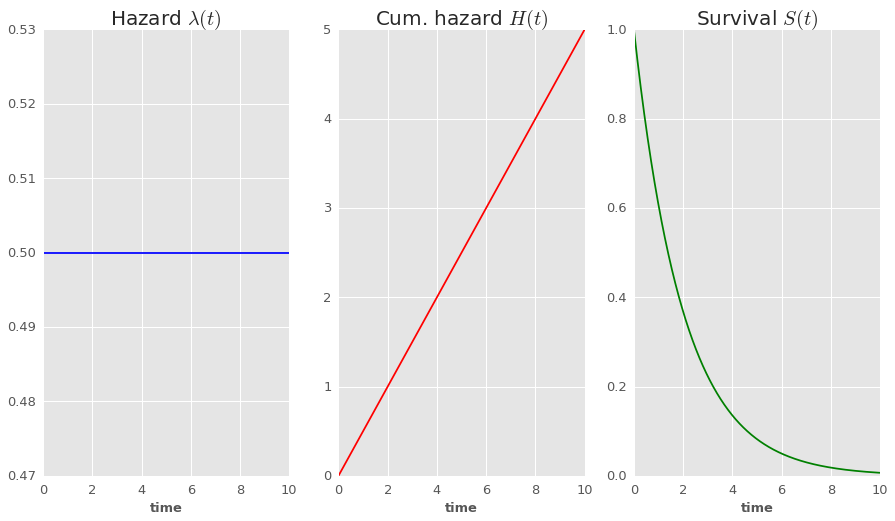In :
## prep simulate-data example under Exponential model
def plot_cum_survival(t, event):
# at each time t, calculate the cumulative survival
cum_survival = survival_table_from_events(t, event)
cum_survival.reset_index(0, inplace=True)
cum_survival.rename(columns = {'event_at': 't'}, inplace=True)
cum_survival['Survival'] = cum_survival['at_risk']/max(cum_survival['at_risk'])
# create figure
fig = plt.figure()
_ = plt.plot(cum_survival.t, cum_survival.Survival, 'rs')
_ = plt.xlabel('time')
return(cum_survival)


We can also simulate survival times for a population under this fairly simple model.

In :
# define a function to simulate data
def simulate_exp_survival_data(N, censor_time, rate):
## simulate true lifetimes (t) according to exponential model
sample_data = pd.DataFrame({
'true_t': np.random.exponential((1/rate), size=N)
})
## censor observations at censor_time
sample_data['t'] = np.minimum(sample_data['true_t'], censor_time)
sample_data['event'] = sample_data['t'] >= sample_data['true_t']
return(sample_data)

In :
# simulate data assuming constant hazard over time of 0.5
df = simulate_exp_survival_data(N = 100, censor_time = 6, rate = 0.5)

Out:
true_t t event
0 0.137413 0.137413 True
1 4.310870 4.310870 True
2 1.685407 1.685407 True
3 2.228120 2.228120 True
4 0.632862 0.632862 True
In :
# plot lifelines for this simulated sample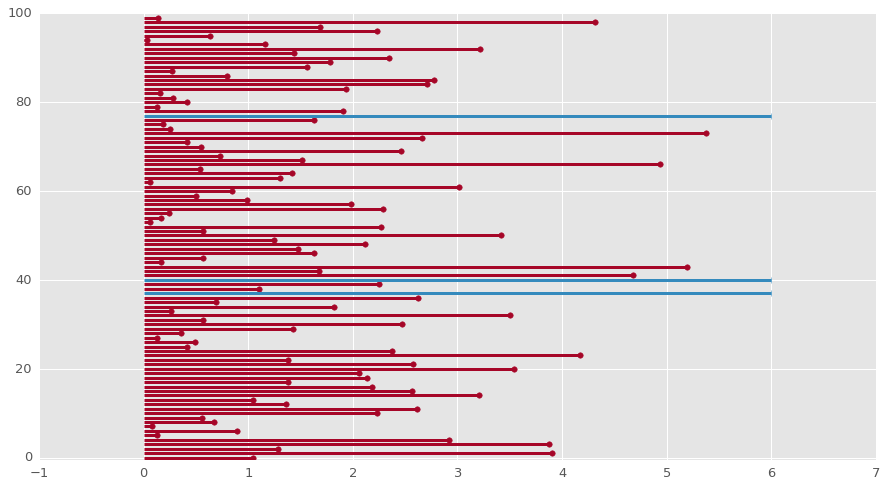In :
# calculate number of people surviving at each time T
# plot Survival to time t given simulated data
a = plot_cum_survival(df.t, df.event)
_ = plt.title('Simulated survival with $\lambda={}$'.format(0.5), size=20)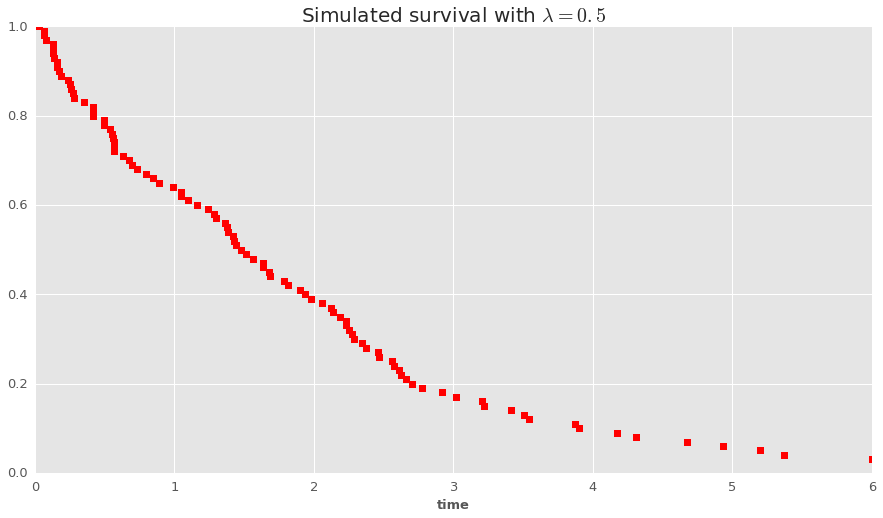Now, overlay computed Survival from simulated data with estimated c.d.f

In :
a = plot_cum_survival(df.t, df.event)
## overlay c.d.f. estimate as exp(-at)

test = pd.DataFrame([{
't': t,
'Estimated Surv': np.exp(-1 * 0.5 * t)
} for t in np.linspace(0, 10, num=100)])
_ = plt.plot(test.t, test['Estimated Surv'], 'b')
_ = plt.legend()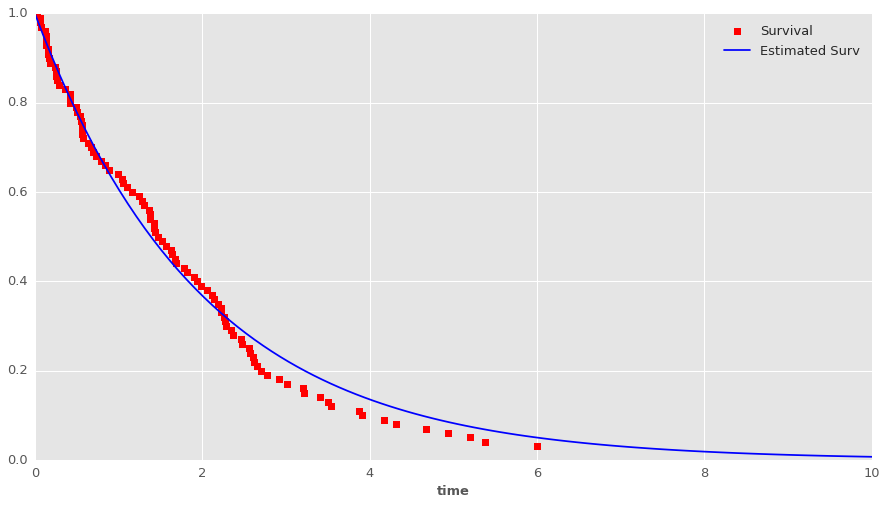# the likelihood¶

The data for survival analysis typically constitute a set of observed pairs: [t, status] for each subject, where

• t is the survival time (last time a subject was observed alive)
• status is a binary (T/F or 1/0) indicator for whether the failure event occurred at time t.

The likelihood for an observation $i$ with time $t_i$ and status = 1 (DECEASED) will reflect the joint probability of surviving to time $t$ and having an event at time $t$:

$$L_i = f(t_i) = S(t_i)\lambda(t_i)$$

For an observation that is censored at time $t$, we only have a contribution to the Survival function :

$$L_i = f(t_i) = S(t_i)$$

Most of the time, we are interested in modeling the impact of covariates on these outcomes.

The typical starting point is to make what's called the Proportional Hazards assumption.

IE:

$$h_X(t_i) = e^{\beta X}h(t_i)$$

For example, in the context of our previous example of Survival times simulated according to the Exponential model, we have:

In :
## prep simulate-data example under Exponential model
def plot_cum_survival_X(df):
# at each time t, calculate the cumulative survival
data_true = df.loc[df['X'] == True,]
cumsurv_true = survival_table_from_events(data_true.t, data_true.event)
cumsurv_true.reset_index(0, inplace=True)
cumsurv_true['X'] = True
cumsurv_true.rename(columns = {'event_at': 't'}, inplace=True)
cumsurv_true['Survival'] = cumsurv_true['at_risk']/max(cumsurv_true['at_risk'])

data_false = df.loc[df['X'] == False,]
cumsurv_false = survival_table_from_events(data_false.t, data_false.event)
cumsurv_false.reset_index(0, inplace=True)
cumsurv_false['X'] = False
cumsurv_false.rename(columns = {'event_at': 't'}, inplace=True)
cumsurv_false['Survival'] = cumsurv_false['at_risk']/max(cumsurv_false['at_risk'])

cum_survival = cumsurv_true.append(cumsurv_false)
# create figure
fig = plt.figure()
_ = sb.lmplot(data = cum_survival, x='t', y='Survival', hue='X', fit_reg=False)

In :
# define a function to simulate data
def simulate_exp_survival_data_X(N, censor_time, rate, beta):
## simulate true lifetimes (t) according to exponential model
sample_data = pd.DataFrame({
'X' : [np.random.uniform()>0.5 for n in np.zeros(N)],
'baseline_hazard': np.repeat(rate, repeats=N),
})
sample_data['hazard'] = np.exp(beta*sample_data['X'])*sample_data['baseline_hazard']
sample_data['true_t'] = sample_data.apply(lambda row: np.random.exponential(scale = 1/row['hazard']), axis = 1)
## censor observations at censor_time
sample_data['t'] = np.minimum(sample_data['true_t'], censor_time)
sample_data['event'] = sample_data['t'] >= sample_data['true_t']
return(sample_data)

In :
df = simulate_exp_survival_data_X(N=100, censor_time=6, rate=0.9, beta=0.2)
plot_cum_survival_X(df)

<matplotlib.figure.Figure at 0x7f4b5dd7d410>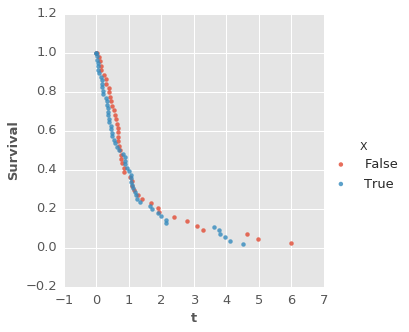In :
# fit exponential model to these data
import stanity
import survivalstan

stan/exp_survival_model.stan
stan/pem_survival_model_unstructured.stan
stan/pem_survival_model_gamma.stan
stan/pem_survival_model_randomwalk_bspline_est_xi.stan
stan/pem_survival_model_randomwalk.stan
stan/pem_survival_model_randomwalk_bspline.stan
stan/weibull_survival_model.stan

In :
weib_model = survivalstan.fit_stan_survival_model(df = df,
formula = '~ X',
event_col = 'event',
time_col = 't',
model_code = survivalstan.models.weibull_survival_model,
chains = 4,
iter = 5000,
make_inits = survivalstan.make_weibull_survival_model_inits,
model_cohort = 'exp simulated, weibull model'
)

NOT reusing model.
Ran in 66.599 sec.

/mnt/ssd0/env/local/lib/python2.7/site-packages/stanity/psis.py:228: FutureWarning: elementwise comparison failed; returning scalar instead, but in the future will perform elementwise comparison
elif sort == 'in-place':
/mnt/ssd0/env/local/lib/python2.7/site-packages/stanity/psis.py:246: VisibleDeprecationWarning: using a non-integer number instead of an integer will result in an error in the future
bs /= 3 * x[sort[np.floor(n/4 + 0.5) - 1]]

In :
sb.boxplot(data=weib_model['coefs'], x = 'value', y = 'variable')
plt.title('Posterior estimates of model coefficients, simulated data', size = 15)

Out:
<matplotlib.text.Text at 0x7f4b8145a150>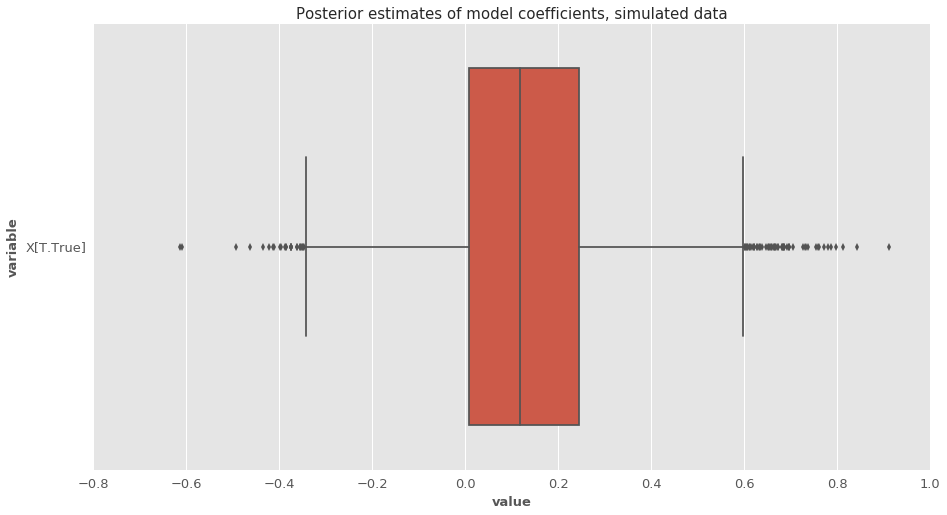In :
## try with exponential baseline hazard
exp_model = survivalstan.fit_stan_survival_model(df = df,
model_code = models['exp_survival_model.stan'],
formula = '~ X',
model_cohort = 'exp simulated, exp model',
event_col = 'event',
time_col = 't',
chains = 4,
iter = 5000,
)

NOT reusing model.
Ran in 55.863 sec.

In :
survivalstan.utils.plot_coefs(models = [exp_model, weib_model])
plt.title('Posterior estimates of model coefficients, simulated data', size = 15)

Out:
<matplotlib.text.Text at 0x7f4b5d6bb4d0>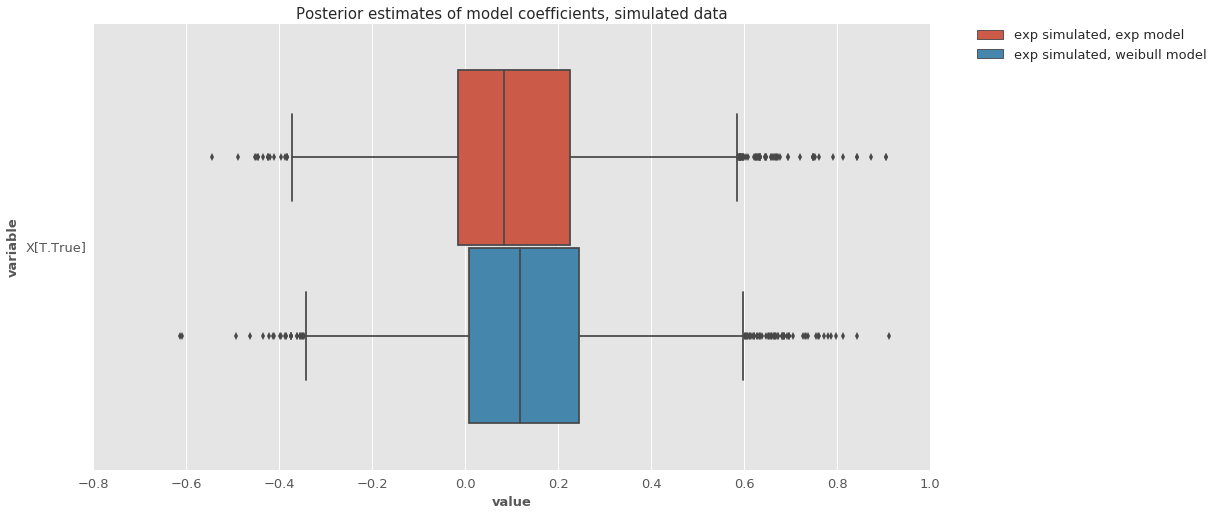In :
stanity.loo_compare(weib_model['loo'], exp_model['loo'])

Out:
{'diff': 0.39328730440969473, 'se_diff': 0.52756534110618469}

Posterior predictive checking

Basic strategy:

1. Compute posterior estimates of y, assuming no censoring
2. For each iteration, summarize proportion of population surviving to time t
3. Summarize survival over iterations (requires selecting desired summary timepoints)
In :
## example for exponential model - extract yhat_uncens observations
posterior_preds = survivalstan.utils.extract_params_long(models=[exp_model], element='yhat_uncens')
posterior_preds.rename(columns = {'variable': 'index', 'value': 'ppred_t'}, inplace=True)
posterior_preds['ppred_event'] = True

Out:
iter index ppred_t model_cohort ppred_event
0 0 0 0.129399 exp simulated, exp model True
1 1 0 1.745296 exp simulated, exp model True
2 2 0 0.240160 exp simulated, exp model True
3 3 0 0.167429 exp simulated, exp model True
4 4 0 1.361147 exp simulated, exp model True
In :
##  for each iteration, summarize cumulative survival at each observed timepoint
posterior = posterior_preds.groupby(['iter']).apply(
lambda row: survival_table_from_events(event_observed=row['ppred_event'], death_times=row['ppred_t']),
)

In :
## rename variables
## (should really be done by model cohort, but ok here since max is same for both groups)
posterior['Survival'] = posterior['at_risk']/max(posterior['at_risk'])
posterior.reset_index(inplace=True)

Out:
iter event_at removed observed censored entrance at_risk Survival
0 0 0.000000 0.0 0.0 0.0 100.0 100.0 1.00
1 0 0.001416 1.0 1.0 0.0 0.0 100.0 1.00
2 0 0.002300 1.0 1.0 0.0 0.0 99.0 0.99
3 0 0.025914 1.0 1.0 0.0 0.0 98.0 0.98
4 0 0.033246 1.0 1.0 0.0 0.0 97.0 0.97
In :
## define time intervals at which we want to summarize estimates
time_bins = np.linspace(0, 10, 100)
posterior['time_interval'] = np.digitize(posterior['event_at'], time_bins)
posterior['interval_t'] = time_bins[posterior['time_interval']-1].astype(float)

Out:
iter event_at removed observed censored entrance at_risk Survival time_interval interval_t
0 0 0.000000 0.0 0.0 0.0 100.0 100.0 1.00 1 0.0
1 0 0.001416 1.0 1.0 0.0 0.0 100.0 1.00 1 0.0
2 0 0.002300 1.0 1.0 0.0 0.0 99.0 0.99 1 0.0
3 0 0.025914 1.0 1.0 0.0 0.0 98.0 0.98 1 0.0
4 0 0.033246 1.0 1.0 0.0 0.0 97.0 0.97 1 0.0
In :
## for each interval, take last observation in the time interval
ppsummary = posterior.copy()
ppsummary = posterior.sort_values(['iter','time_interval','event_at']).groupby([
'iter','time_interval'], as_index=False).last()

Out:
iter time_interval event_at removed observed censored entrance at_risk Survival interval_t
0 0 1 0.096138 1.0 1.0 0.0 0.0 89.0 0.89 0.00000
1 0 2 0.200744 1.0 1.0 0.0 0.0 81.0 0.81 0.10101
2 0 3 0.293032 1.0 1.0 0.0 0.0 74.0 0.74 0.20202
3 0 4 0.397739 1.0 1.0 0.0 0.0 67.0 0.67 0.30303
4 0 5 0.491911 1.0 1.0 0.0 0.0 61.0 0.61 0.40404
In :
## summarize over intervals
sb.boxplot(data = ppsummary,
x = 'time_interval',
y = 'Survival',
fliersize=0)

Out:
<matplotlib.axes._subplots.AxesSubplot at 0x7f4b5d6b7690>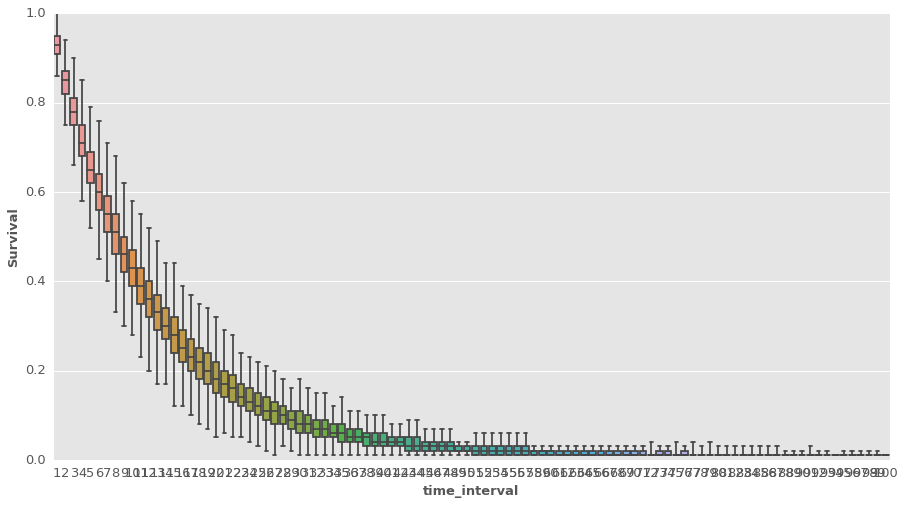In :
def sum_percentiles(df, percentile, by=['time_interval'], keep_cols = ['time_interval', 'interval_t','Survival']):
pdata = df.groupby(by, as_index=False).agg(lambda x: np.percentile(x, percentile))
pdata = pdata.loc[:, keep_cols]
return(pdata)

p10 = sum_percentiles(ppsummary, percentile=10)
p90 = sum_percentiles(ppsummary, percentile=90)

ppsummary2 = pd.merge(p10, p90, on=['time_interval','interval_t'], suffixes = ['_p10','_p90'])

Out:
time_interval interval_t Survival_p10 Survival_p90
0 1 0.00000 0.89 0.96
1 2 0.10101 0.80 0.90
2 3 0.20202 0.72 0.84
3 4 0.30303 0.65 0.78
4 5 0.40404 0.58 0.72
In :
plot_cum_survival(event=df.event, t=df.t)
_ = plt.fill_between(ppsummary2.interval_t,
ppsummary2.Survival_p10,
ppsummary2.Survival_p90,
where= ppsummary2.Survival_p90 >=  ppsummary2.Survival_p10,
facecolor='gray')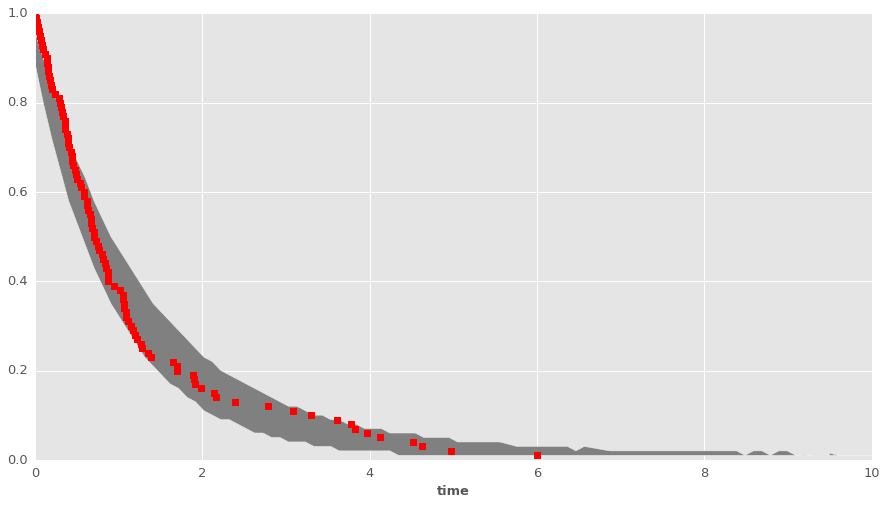These checks can be useful, in two ways:

1. In practice, we don't know the true shape of the baseline hazard.
2. Also, it can help uncover problems in the process of data-simulation and/or estimation.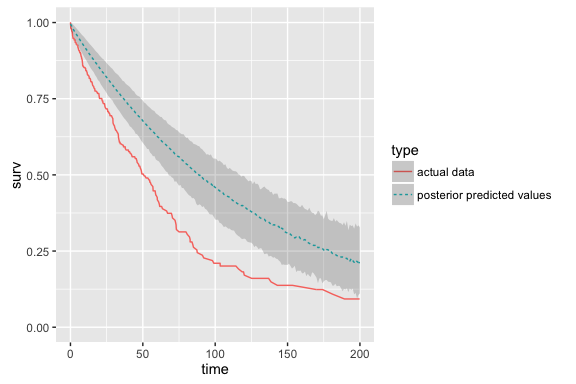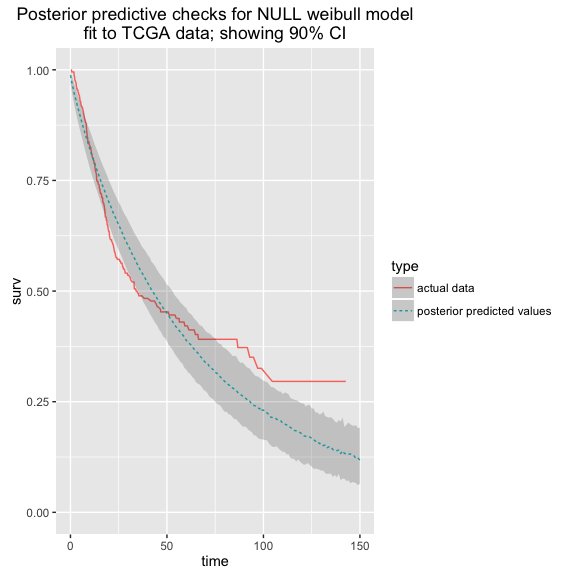## Modeling the baseline hazard¶

There are a variety of methods used to model the baseline hazard.

Parametric:

• Gamma
• exponential
• Weibull

Semi- or non-parametric:

• Gamma, Weibull or exponential priors on hazards
• Gamma-process priors
• random-walk priors
• unconstrained piecewise hazard

Often we want to find a way to fit baseline hazards more flexibly, in order to better understand covariate effects.

One approach to this is to use a "binned likelihood".

In :
unique_failure_times=df['t'].unique()
for ftime in unique_failure_times:
plt.vlines(ftime, 0, 100, lw=0.5, linestyles="--")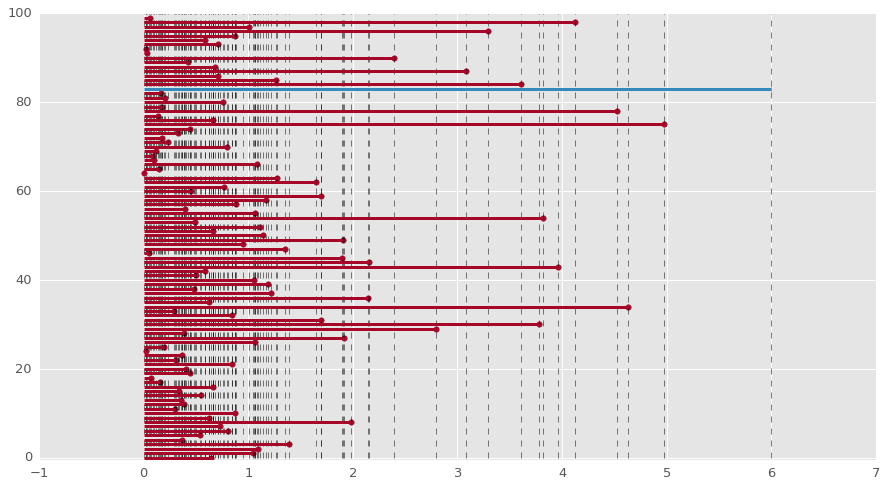In :
## transform data
dflong = survivalstan.prep_data_long_surv(df=df.reset_index(), event_col='event', time_col='t')

Out:
index X baseline_hazard hazard true_t t event key end_time end_failure
3535 35 True 0.9 1.099262 0.004904 0.004904 True 1 0.004904 True
7535 75 True 0.9 1.099262 0.018886 0.018886 True 1 0.004904 False
7575 75 True 0.9 1.099262 0.018886 0.018886 True 1 0.018886 True
775 7 False 0.9 0.900000 0.023054 0.023054 True 1 0.018886 False
707 7 False 0.9 0.900000 0.023054 0.023054 True 1 0.023054 True

### unstructured baseline hazard¶

Now that we have prepared our data in the right format, we are ready to fit the PEM model.

The first model we will consider is what I call an "unstructured" baseline hazard, since each timepoint is estimated somewhat independently from one another.

We have an overall average hazard, called log_baseline_mu, and the hazard for each time period is estimated as an offset from this overall mean.

Here is our parameters block:

parameters {
vector[T] log_baseline_raw; // unstructured baseline hazard for each timepoint t
vector[M] beta;         // beta for each covariate
real<lower=0> baseline_sigma;
real log_baseline_mu;
}

and, our transformed parameters block:

transformed parameters {
vector[N] log_hazard;
vector[T] log_baseline;     // unstructured baseline hazard for each timepoint t

log_baseline = log_baseline_raw + log_t_dur;  // adjust for duration of each time period

for (n in 1:N) {
log_hazard[n] = log_baseline_mu + log_baseline[t[n]] + x[n,]*beta;
}
}

Our model block simply puts a prior on the log_baseline_mu & log_baseline_raw parameters.

model {
beta ~ cauchy(0, 2);
event ~ poisson_log(log_hazard);
log_baseline_mu ~ normal(0, 1);
baseline_sigma ~ normal(0, 1);
log_baseline_raw ~ normal(0, baseline_sigma);
}
In :
## fit unstructured-hazard model to these data
pem_unstr = survivalstan.fit_stan_survival_model(df = dflong,
formula = '~ X',
event_col = 'end_failure',
timepoint_end_col = 'end_time',
sample_col = 'index',
model_code = models['pem_survival_model_unstructured.stan'],
chains = 4,
iter = 1000,
model_cohort = 'exp simulated, unstructured hazard',
)

NOT reusing model.
Ran in 97.042 sec.


Not surprisingly, we find little evidence that the hazard varies across timepoints.

In :
survivalstan.utils.plot_coefs([pem_unstr], element='baseline_raw')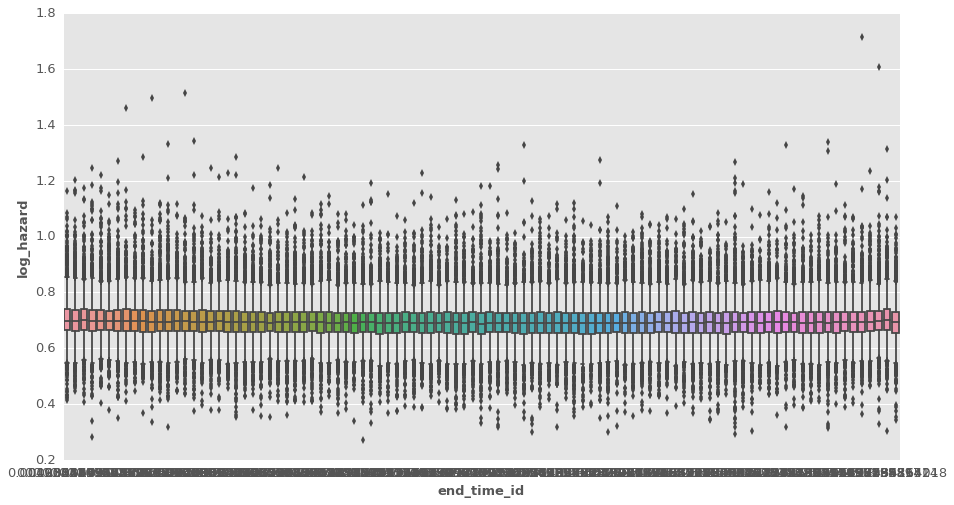How does the coefficient estimate from this unstructured model compare to that from our Weibull & Exponential models?

In :
survivalstan.utils.plot_coefs([pem_unstr, weib_model, exp_model])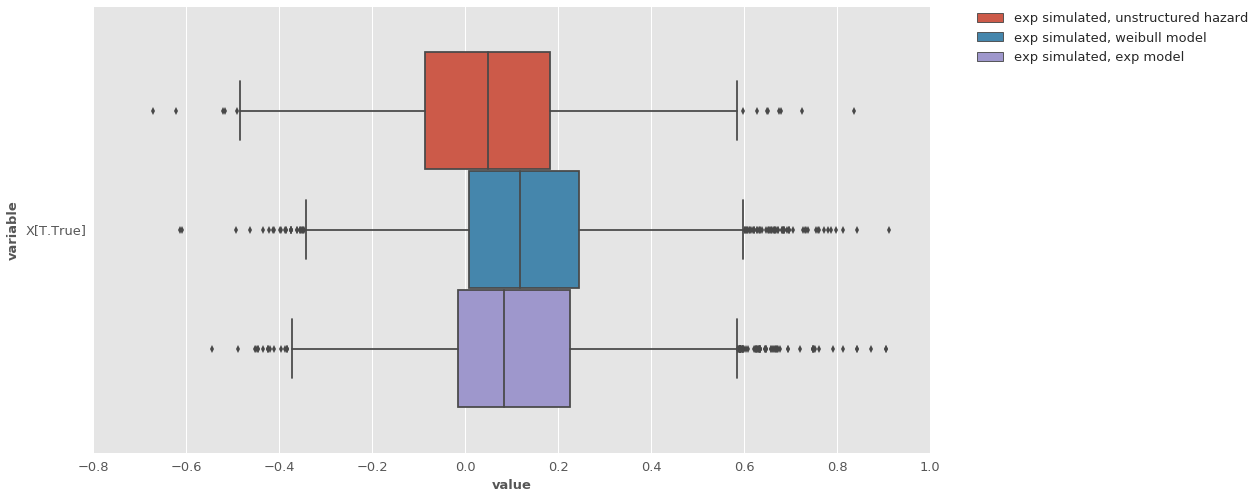### Random-walk baseline hazard¶

An alternative to the unstructured baseline hazard is to use what's called a random walk baseline hazard.

This is a slight modification from the previous baseline hazard, such that each time period has a hazard is an offset from (i.e. borrows information from) the hazard estimate from the the previous time period.

There are several ways to specify a random walk, such as by imposing a correlation structure on the estimates of baseline hazards. Here we have written the baseline hazard very explicitly in our model block, as follows:


model {
# [ ... some code omitted ... ]
log_baseline_raw ~ normal(0, 1);
for (i in 2:T) {
log_baseline_raw[i] ~ normal(log_baseline_raw[i-1], baseline_sigma);
}
}
In :
## fit randomwalk-prior model to these data
pem_randomwalk = survivalstan.fit_stan_survival_model(df = dflong,
formula = '~ X',
event_col = 'end_failure',
timepoint_end_col = 'end_time',
sample_col = 'index',
model_code = models['pem_survival_model_randomwalk.stan'],
chains = 1,
iter = 5000,
model_cohort = 'exp simulated, random walk prior hazard'
)

NOT reusing model.
Ran in 552.588 sec.


The resulting baseline_raw hazard estimates from this model reflect the structure we imposed on the sample -- recall that we only have a sample size of n=100.

In this model, our baseline hazard has an overall mean (log_baseline_mu) and the deviance from that mean is allowed to vary from one timepoint to the next.

Let's see what this looks like in practice:

In :
survivalstan.utils.plot_coefs([pem_randomwalk], element='baseline_raw')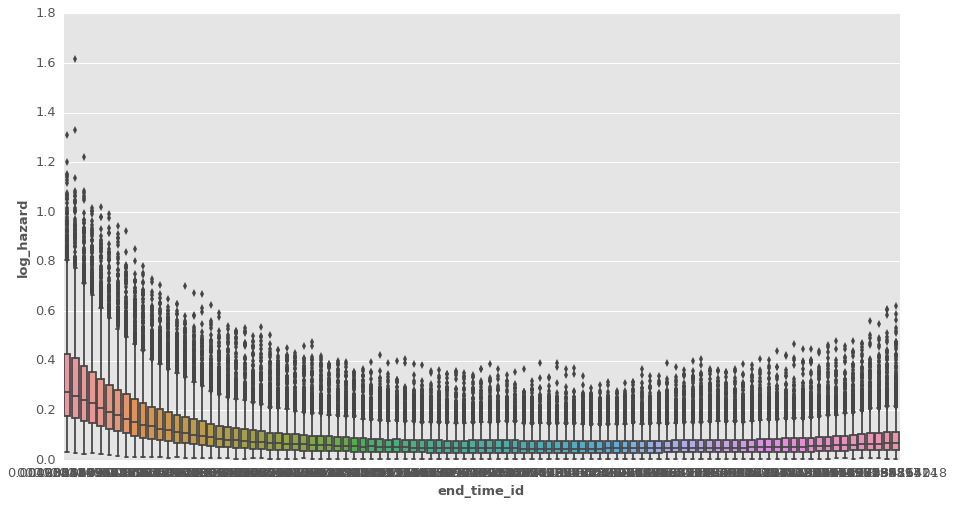Now, a perhaps the more important question is how this variance in our baseline hazard impacts our coefficient estimates.

In :
survivalstan.utils.plot_coefs([pem_unstr, pem_randomwalk, weib_model, exp_model])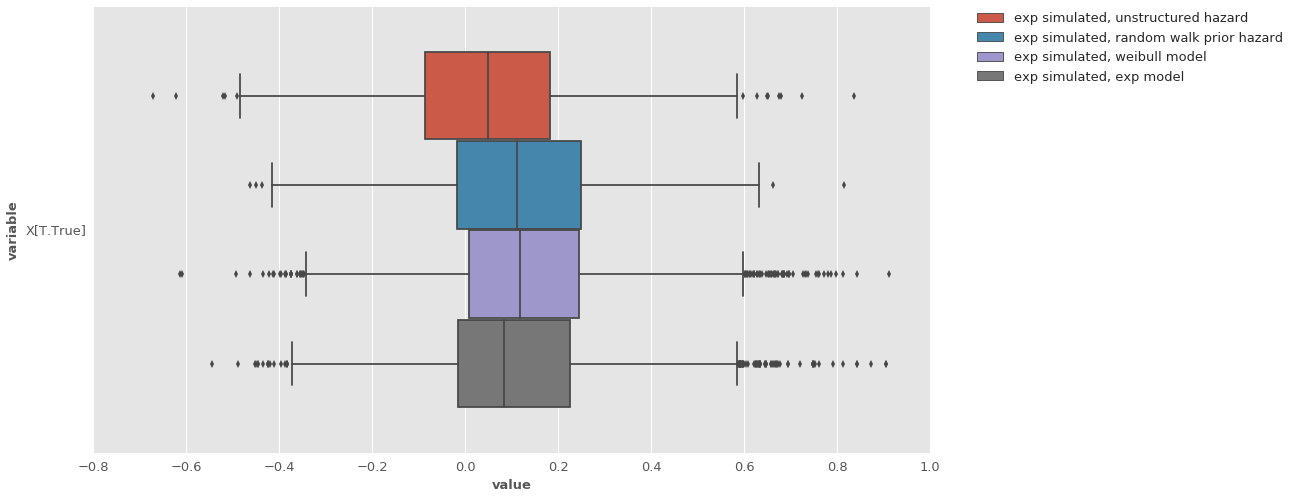In general, it appears that these models behave very similarly -- which is good.

Let's compare to the "True" beta (the value used to simulate the data) of 0.2.

In :
survivalstan.utils.plot_coefs([pem_unstr, pem_randomwalk, weib_model, exp_model])
plt.vlines(0.2, -200, 200, linestyles='--')

Out:
<matplotlib.collections.LineCollection at 0x7f4b759f0990>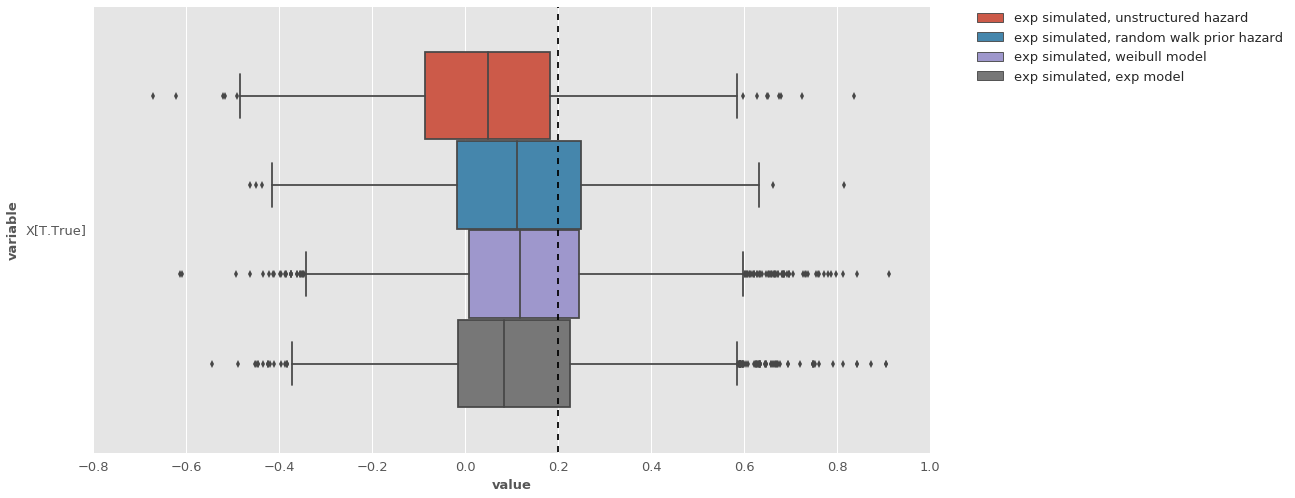Compare model fit with unstructured baseline hazard to that with random walk. Is there a difference between the two models according to PSIS-LOO?

In :
stanity.loo_compare(pem_unstr['loo'], pem_randomwalk['loo'])

Out:
{'diff': 6.7186238987099252, 'se_diff': 5.0038264166095843}

For this model, we wouldn't expect a strong difference between these two models, since the true hazard we used to simulate the data did not vary over time. In practice, hazards can and sometimes do vary over time.

One of the challenges of survival analysis in practice, particularly with small sample sizes, is to stabilize the estimates of the baseline hazard, since our inferences for coefficient effects are multiplicative on this hazard.

In a companion notebook, we work through a process for analyzing data from TCGA, focusing on the BLCA cohort.

In [ ]: